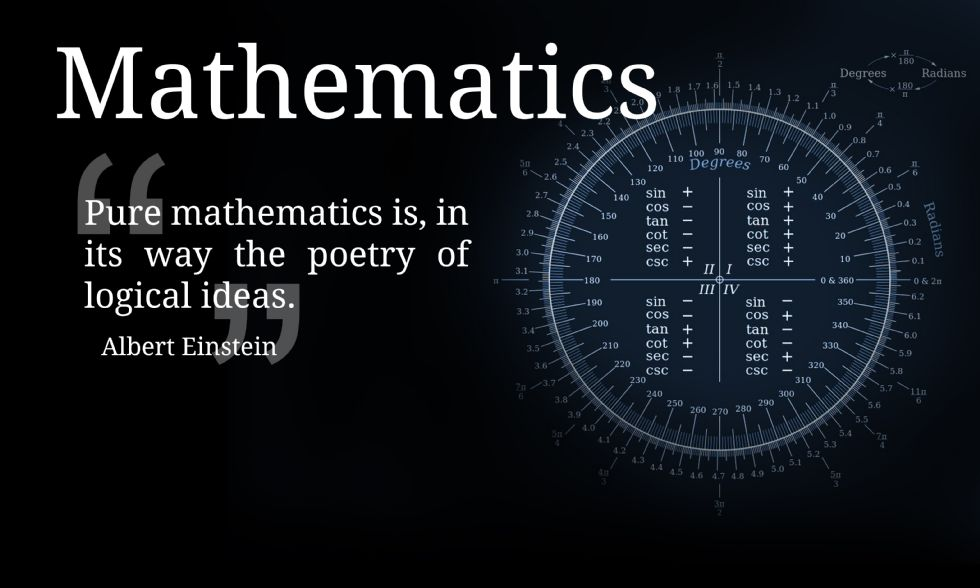Water Orton Road, Castle Bromwich, Birmingham. B36 9HF

Telephone:0121 748 0400

Fax:0121 748 0414

Contact:Mr Cox

# Year 8### Year 8 Foundation and Higher

 Assessment Cycle Topics covered 1 Fractions, decimals and percentages Simplifying ratio Sharing in a ratio Recipes Interpreting scales Measure angles Scale drawings Finding enlargement scale factor Enlarging a shape by a scale factor, including fractional Probability of an event Sample spaces Experimental probability 2 Types of data Constructing graphs Averages Averages from a frequency table Draw and interpret pie charts Coordinates Scatter graphs Stem and leaf diagrams Percentages of amounts Percentage increase and decrease Fraction of amounts 3 Area and perimeter Area and circumference of circles Constructing triangles Pythagoras’ theorem Simplifying algebra Expanding brackets Factorising expressions Substitution Solving equations Summer Drawing straight line graphs Drawing quadratics Proportion